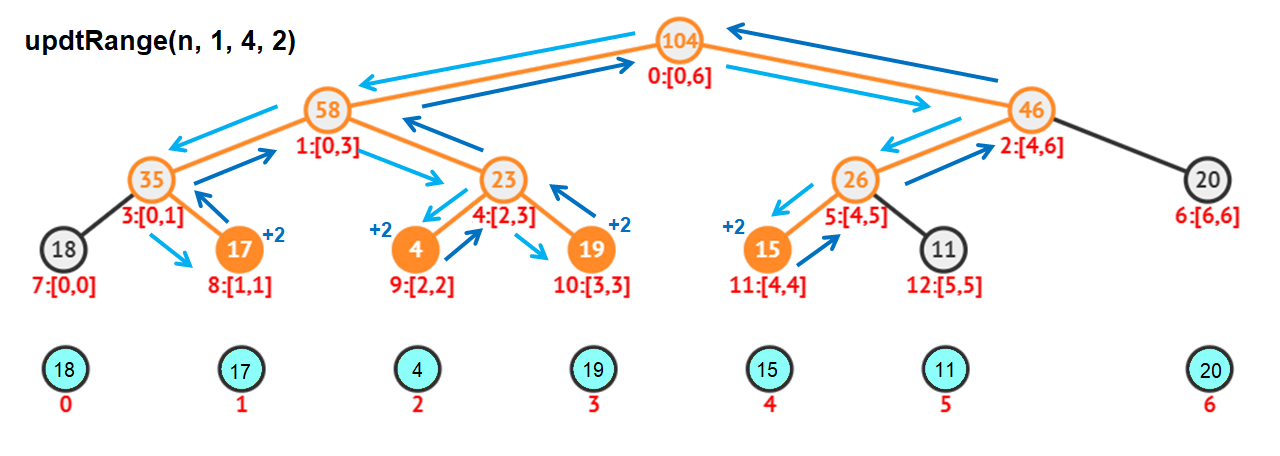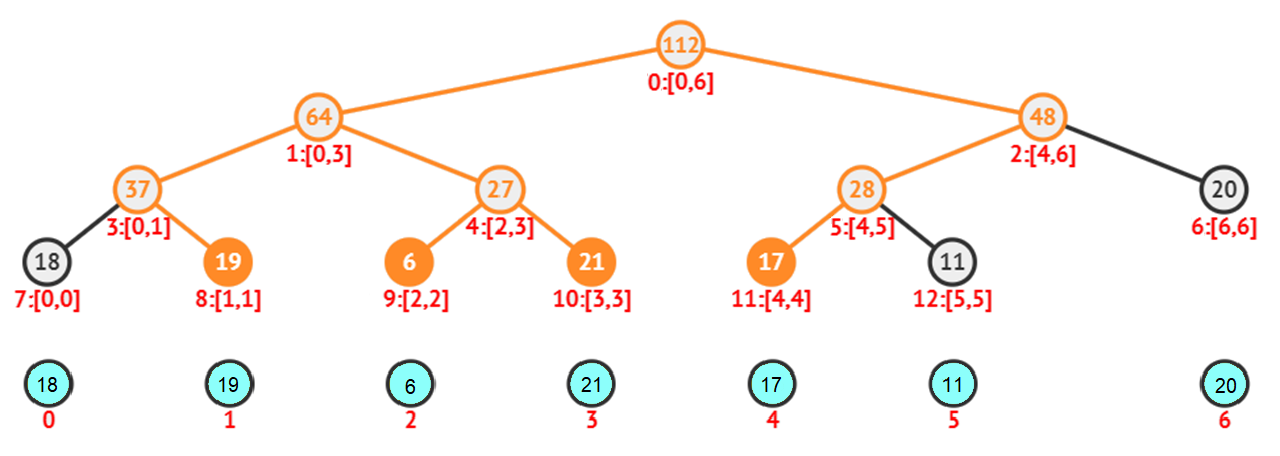# Lazy Propagation in Segment Tree using C++

In this tute, we will discuss Lazy propagation in Segment tree using C++. If you haven’t gone through the basics of implementing segment trees, head straight to sum of range in segment tree before this.

### Range updates in Segment Tree:

On the other post on updates in the Segment Tree, we discussed how range updates work on Segment trees. The general idea is that we start from the root of the segment tree and reach the leaves within the given range, change them, return back to the root. In this process, we change a few internal nodes as well.

Let’s see the algorithm we used in the other post:

2. Then, at each intermediate node, enter into those child nodes which are within the range.
3. If the expected leaves are reached, update them and return to the root.
4. While returning to the root, recalculate all the intermediate nodes.

Below is the code snippet used in the other post:

```/* Function to update a range of array elements in the Segment
Tree. strt and end are the range in the array. val is the value
to be added. idx is index of node in segment tree. l and r are
the range covered by the node */
void updtRange(int strt, int end, int val, int l, int r, int idx)
{
// Completly ouside the desired range
if(end < l || r < strt || l>r)
return;
// Leaf node reached
if(l == r)
{
tree[idx] += val;  // Update the leaves
return;
}
int mid = (l + r) / 2;
updtRange(strt, end, val, l, mid, 2*idx+1);
updtRange(strt, end, val, mid+1, r, 2*idx+2);
// Update the intermediate nodes
tree[idx] = tree[2*idx+1] + tree[2*idx+2];
}```After update,Here, we can see that if an intermediate node is completely within the range such as node 4:[2,3], all its child nodes are updated as well, that is, all the elements in the range [2,3] are updated. This important observation is key for the optimization done on range updates.

## Lazy Propagation in Segment Tree:

Optimization of the range updates by postponing updates at some nodes is referred to as Lazy propagation.

Let’s look into an example. Try to imagine the top view of the tree and enter into the desired nodes only. In the update image, we can see that node 4 in the segment tree having a range [2, 3] has both its children being updated. Later, if a query is done for the range [2, 3], the traversal stops at node 4 because the node is entirely within the desired range. In this scenario, the update operation could’ve been done at node 4 and its children could’ve been updated later. This is because of the fact that in the query operation, we are accessing the node 4 only. What if we could do this for other cases as well?

We can create a new tree named lazy representing the lazy tree. The size and structure of the lazy tree are similar to the original segment tree. The idea here is that while doing update operation if we encounter an intermediate node which is entirely within the range, let’s update that node in the segment tree by using the simple formula,

`idx:[l,r] => tree[idx] += ( r - l + 1 ) * val;`

Then, postpone the updates of its children by saving those update values in the lazy tree.

The lazy tree is initialized with 0 at the start. If lazy[idx] is 0, then there are no pending updates. Otherwise, all the elements in its range are to be updated with the value lazy[idx].

#### Algorithm:

```updateSegTree(strt, end, val):
1. If lazy[idx] != 0 then
Add the pending update to the current segment tree node
If the node has children, postpone the updates to its children
2. If the current node lies completely within the range then
Update the current node
Postpone the update to its children by adding to lazy nodes of the children
Else
Enter into the child that is within the range
Update the node by recalculating with its child node values after updating
```

## Query Operation: Segment Tree

While in the process of optimizing the range updates, we shouldn’t forget that it’d affect the query method as well. Since we are postponing the updates at a few nodes, we may end up forgetting about those pending updates if we use the old method. So, we must alter the query function as well.

#### Algorithm:

```getSum(strt, end):
1. If lazy[idx] != 0 then
Add the pending update to the current segment tree node
If the node has children, postpone the updates to its children
2. If the current node lies completely within the range then
Return the current node
Else
Enter into the child that is within the range
Update the node by recalculating with its child node values after updating```

### Implementation:

```// C++ program to showLazy propagation in Segment Tree - Sum of range
#include<bits/stdc++.h>
using namespace std;

#define MAX 100010
int tree[MAX], lazy[MAX];
vector<int> ar;

/* Function to display elements of the Segment Tree and
the Lazy tree level by level. */
void showSegTree(int n)
{
int i, j, h = ceil(log2(n));
cout<<"Segment Tree:"<<endl;
for(i=0 ; i<=h ; ++i)
{
for(j=0 ; j<pow(2, i) ; ++j)
cout<<tree[int(pow(2, i)-1 + j)]<<' ';
cout<<endl;
}
cout<<"Lazy Tree:"<<endl;
for(i=0 ; i<=h ; ++i)
{
for(j=0 ; j<pow(2, i) ; ++j)
cout<<lazy[int(pow(2, i)-1 + j)]<<' ';
cout<<endl;
}
}

/* Function to construct the Segment Tree recursively.
At each node, if it is not the leaf, two children are
processed further. Otherwise, the leaf is assigned an
array alement. strt and end are range in the array,
idx is the index used in the segment tree */
int constSegTree(int strt, int end, int idx)
{
// Initialize all lazy tree nodes with 0
lazy[idx] = 0;

// Saves tree[idx] = ar[strt] and then returns tree[idx]
if(strt == end)
return tree[idx] = ar[strt];

int mid = (strt + end) / 2;
return tree[idx] = constSegTree(strt, mid, 2*idx+1) +
constSegTree(mid+1, end, 2*idx+2);
}
void constSegTree(int n)
{
constSegTree(0, n-1, 0);
}

/* Function to get the sum of elements of array in the
range [strt..end]. l & r are the range of current node.
indx is the index of node in segment tree */
int getSum(int strt, int end, int l, int r, int idx)
{
// If there's pending update, update the node first
if(lazy[idx] != 0)
{
// Add the pending update to the current node
// [l..r] contains l-r+1 nodes
tree[idx] += (r-l+1)*lazy[idx];

// If the node has children, postpone the updates
// to the children by adding to the their lazy nodes
if(l != r)
{
lazy[2*idx+1] += lazy[idx];
lazy[2*idx+2] += lazy[idx];
}

// Reset the lazy node of current node
lazy[idx] = 0;
}

// If completly outside the range, don't care
if(end < l || r < strt || l > r)
return 0;

// If node is entirely within the range, return the node value
if(strt <= l && r <= end)
return tree[idx];

// Enter its children and process further
int mid = (l + r) / 2;
return getSum(strt, end, l, mid, 2*idx+1) +
getSum(strt, end, mid+1, r, 2*idx+2);
}
int getSum(int n, int strt, int end)
{
return getSum(strt, end, 0, n-1, 0);
}

/* Function to update a particular node of the array in
Segment Tree. pos is the desired index in the array.
val is the value to be added */
void updtSegTree(int strt, int end, int val, int l, int r, int idx)
{
// If there's pending update, update the node first
if(lazy[idx] != 0)
{
// Add the pending update to the current node
// [l..r] contains l-r+1 nodes
tree[idx] += (r-l+1)*lazy[idx];

// If the node has children, postpone the updates
// to the children by adding to the their lazy nodes
if(l != r)
{
lazy[2*idx+1] += lazy[idx];
lazy[2*idx+2] += lazy[idx];
}

// Reset the lazy node of current node
lazy[idx] = 0;
}

// If completly outside the range, don't care
if(end < l || r < strt || l > r)
return;

// If node is entirely within the range
if(strt <= l && r <= end)
{
// Update the current node in Segment tree
tree[idx] += (r-l+1)*val;

// If the node has children, postpone the updates
// of the children by adding to the their lazy nodes
if(l != r)
{
lazy[2*idx+1] += val;
lazy[2*idx+2] += val;
}
}
else
{
// Enter its children and process further
int mid = (l + r) / 2;
updtSegTree(strt, end, val, l, mid, 2*idx+1);
updtSegTree(strt, end, val, mid+1, r, 2*idx+2);

// Recalculate the itermediate nodes after updation
tree[idx] = tree[2*idx+1] + tree[2*idx+2];
}
}
void updtSegTree(int n, int strt, int end, int val)
{
updtSegTree(strt, end, val, 0, n-1, 0);
}

int main()
{
int n = 7;
ar = {18, 17, 1, 19, 15, 11, 20};

constSegTree(n);

cout<<"n = 7, ar = {18, 17, 1, 19, 15, 11, 20}"<<endl;
showSegTree(n);

updtSegTree(n, 0, 4, 2);

cout<<"\nupdtSegTree(n, 0, 4, 2):"<<endl;
showSegTree(n);

cout<<"\nSum of ar[1..4] = "<<getSum(n, 1, 4)<<endl;
showSegTree(n);

return 0;
}```

Output:

```n = 7, ar = {18, 17, 1, 19, 15, 11, 20}
Segment Tree:
101
55 46
35 20 26 20
18 17 1 19 15 11 0 0
Lazy Tree:
0
0 0
0 0 0 0
0 0 0 0 0 0 0 0

updtSegTree(n, 0, 4, 2):
Segment Tree:
111
63 48
35 20 28 20
18 17 1 19 17 11 0 0
Lazy Tree:
0
0 0
2 2 0 0
0 0 0 0 0 0 0 0

Sum of ar[1..4] = 60
Segment Tree:
111
63 48
39 24 28 20
20 19 1 19 17 11 0 0
Lazy Tree:
0
0 0
0 0 0 0
0 0 2 2 0 0 0 0```

Bonus: If you still find it difficult to understand the implementation of lazy propagation in segment tree, remove all comments in the code and start tracing for your inputs.